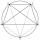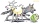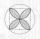# Wiring

Conduit has a cross section 482 mm. Maybe put it into 6 conductors with a cross section S2 \$mm2?

Result

#### Solution:

$r_1 = \sqrt{ \dfrac{ 48}{ \pi}} = 3.91 \ \text{mm} \ \\ r_2 = \sqrt{ \dfrac{ 5.4}{ \pi}} = 1.31 \ \text{mm} \ \\ \ \\ 2\cdot r_1 < 6 \cdot r_2 \ \\$

Leave us a comment of this math problem and its solution (i.e. if it is still somewhat unclear...):Be the first to comment!## Next similar math problems:

1. Pipe cross sectionThe pipe has an outside diameter 1100 mm and the pipe wall is 100 mm thick. Calculate the cross section of this pipe.
2. AceThe length of segment AB is 24 cm and the point M and N divided it into thirds. Calculate the circumference and area of this shape.
3. 10 piecesHow to divide the circle into 10 parts (geometrically)?
4. Roof tilesThe roof has a trapezoidal shape with bases of 15 m and 10 m, height of roof is 4 meters. How many tiles will need if on 1 m2 should be used 8 tiles?
5. An equilateralAn equilateral triangle with a side 10 m represents a wooden platform standing in a lawn. A goat is tied to a corner with a 15 m rope. What is the maximum amount of grazing area available to the goat?
6. QuatrefoilCalculate area of the quatrefoil which is inscribed in a square with side 6 cm.In six baskets, the seller has fruit. In individual baskets, there are only apples or just pears with the following number of fruits: 5,6,12,14,23 and 29. "If I sell this basket," the salesman thinks, "then I will have just as many apples as a pear." Which
8. Regular octagonDraw the regular octagon ABCDEFGH inscribed with the circle k (S; r = 2.5 cm). Select point S' so that |SS'| = 4.5 cm. Draw S (S '): ABCDEFGH - A'B'C'D'E'F'G'H'.
9. Circular lawnAround a circular lawn area is 2 m wide sidewalk. The outer edge of the sidewalk is curb whose width is 2 m. Curbstone and the inner side of the sidewalk together form a concentric circles. Calculate the area of the circular lawn and the result round to 1
10. Theorem proveWe want to prove the sentence: If the natural number n is divisible by six, then n is divisible by three. From what assumption we started?
11. Diameters of circlesHow many percent of the area of a larger circle is a smaller circle if the smaller circle has a diameter 120 mm and a larger one has a diameter 300 mm?
12. The diceWhat is the probability of events that if we throw a dice is rolled less than 6?
13. AntennasIf you give me two antennas will be same. If you give me again your two antenna I have a 5× so many than you. How many antennas have both mans?
14. CandiesIf Alena give Lenka 3 candy will still have 1 more candy. If Lenka give Alena 1 candy Alena will hame twice more than Lenka. How many candies have each of them?
15. Linear systemSolve a set of two equations of two unknowns: 1.5x+1.2y=0.6 0.8x-0.2y=2
16. In the orchardIn the orchard, they planted 25 apple trees, 20 pears, 15 plums and 40 marbles. A strong late frost, however, destroyed a fifth of all new trees. Unfortunately, it was all the trees of one kind of fruit. What is the probability that the plums have died out
17. PowersExpress the expression ? as the n-th power of the base 10.